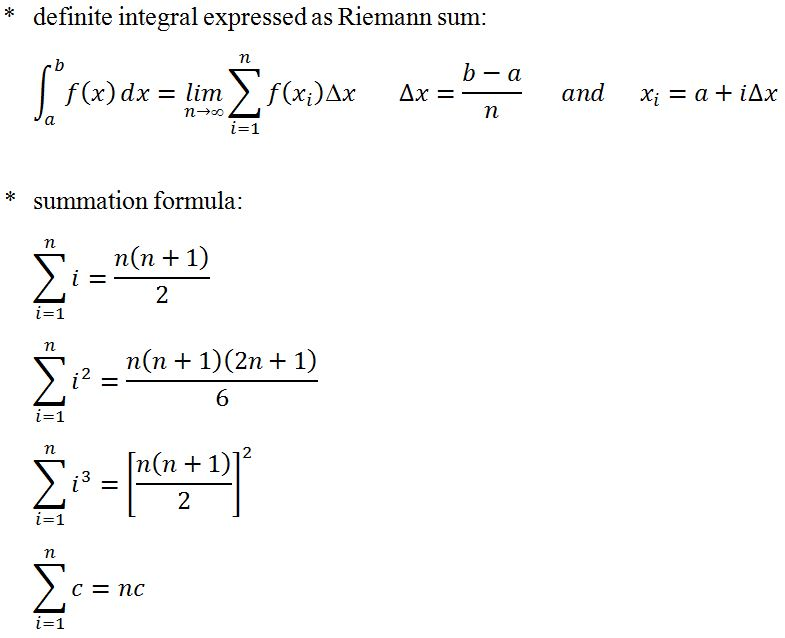# Riemann sum

### Riemann sum

In this lesson, we will learn how to approximate the area under the curve using rectangles. First we notice that finding the area under the curve is easy if the function is a straight line. However, what if it is a curve? We will actually have to approximate curves using a method called "Riemann Sum". This method involves finding the length of each sub-interval (delta x), and finding the points of interest, finding the y values of each point of interest, and then use the find the area of each rectangle to sum them up. There are 3 methods in using the Riemann Sum. First is the "Right Riemann Sum", second is the "Left Riemann Sum", and third is the "Middle Riemann Sum". Lastly, we will look at the idea of infinite sub-intervals (which leads to integrals) to exactly calculate the area under the curve.
Basic Concepts: Riemann sum

#### Lessons• Introduction
Riemann Sum overview:
a)
area under the curve

b)
summation formula

c)
what is "Riemann Sum"?

d)
integrals expressed as Riemann Sum

• 1.
Finding the area under the graph of a function using a graphing calculator.
Consider the function $f\left( x \right) = {x^2}$, $1 \le x \le 3$.
Find the area under the graph of $f$ using a graphing calculator.

• 2.
Finding the area under the graph of a function using the Riemann Sum.
Consider the function $f\left( x \right) = {x^2}$, $1 \le x \le 3$.
Estimate the area under the graph of $f$ using four approximating rectangles and taking the sample points to be:
a)
right endpoints

b)
left endpoints

c)
midpoints

• 3.
Evaluating integrals with a Riemann Sum
Consider the function $f\left( x \right) = {x^2} - 5x + 3$, $2 \le x \le 5$.
a)
Evaluate the Right Riemann sum for $f$ with 6 sub-intervals.

b)
Evaluate $\int_2^5 \left( {{x^2} - 5x + 3} \right)dx$, by finding the Riemann sum for $f$ with $\infty$ intervals.

• 4.
Evaluating Riemann Sum with trapezoids
Consider the function $f\left( x \right) = {x^2}$, $1 \le x \le 3$. Estimate the area under the graph of $f$ using four approximating trapezoids.